Process costing

Process costing is a method of costing used mainly in manufacturing where units are continuously mass-produced through one or more processes. Examples of this include the manufacture of erasers, chemicals or processed food.

In process costing it is the process that is costed (unlike job costing where each job is costed separately). The method used is to take the total cost of the process and average it over the units of production.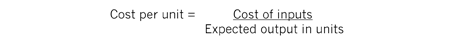Important terms to understand
In a manufacturing process the number of units of output may not necessarily be the same as the number of units of inputs. There may be a loss.

Normal loss
This is the term used to describe normal expected wastage under usual operating conditions. This may be due to reasons such as evaporation, testing or rejects.

Abnormal loss
This is when a loss occurs over and above the normal expected loss. This may be due to reasons such as faulty machinery or errors by labourers.

Abnormal gain
This occurs when the actual loss is lower than the normal loss. This could, for example, be due to greater efficiency from newly-purchased machinery.

Work in progress (WIP)
This is the term used to describe units that are not yet complete at the end of the period. Opening WIP is the number of incomplete units at the start of a process and closing WIP is the number at the end of the process.

Scrap value
Sometimes the outcome of a loss can be sold for a small value. For example, in the production of screws there may be a loss such as metal wastage. This may be sold to a scrap merchant for a fee.

Equivalent units
This refers to a conversion of part-completed units into an equivalent number of wholly-completed units. For example, if 1,000 cars are 40% complete then the equivalent number of completed cars would be 1,000 x 40% = 400 cars. Note: If 1,000 cars are 60% complete on the painting, but 40% complete on the testing, then equivalent units will need to be established for each type of cost. (See numerical example later.)

How to approach process accounting questions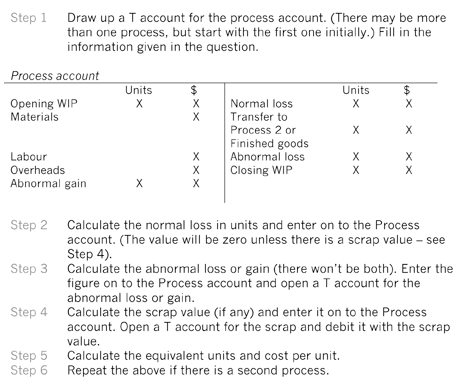Note: Although this proforma includes both losses and WIP, the Paper F2/FMA syllabus specifically excludes situations where both occur in the same process. Therefore, don’t expect to have to complete all of the steps in the questions.

Normal loss example Mr Bean’s chocolate Wiggly bars pass through two processes. The data for the month just ended are: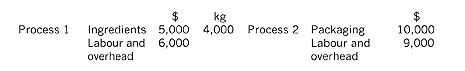Mr Bean allows the staff to eat 5% of the chocolate as they work on Process 1. There was no work in progress at the month end. Prepare the two process accounts and calculate the cost per kg.Workings

1. The staff normally eat 5% of the chocolate, so the normal loss is 4,000 x 5% = 200kg

There is no work in progress or scrap value or abnormal losses or gains, so we can now balance the account to obtain the amounts transferred to Process 2.

2. Number of kg transferred = kg input less normal loss = 4,000 – 200 = 3,800kg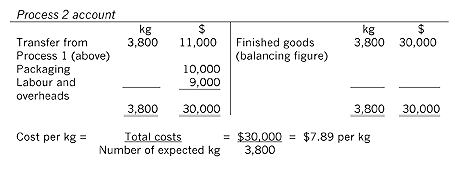Abnormal gain example

There is a heat wave and staff have eaten less chocolate. At the end of Process 1, 3,810 units are transferred to Process 2.Cost of units transferred to Process 2 = \$2.89 x 3,810 = \$11,029 (using \$2.894736842 to avoid rounding differences).
Cost of abnormal gain = \$2.89 x 10 = \$29.
[Remember to open an abnormal gain T account and credit it with \$29]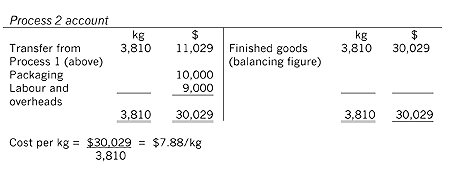Scrap value example
Mr Bean can no longer afford to give his staff 5% of the bars. He decides to offer the bars to his staff at a discount. They pay 40c for every kg that they eat. As a result of this, there is another abnormal gain of 10kg, so 3,810 units are transferred to Process 2.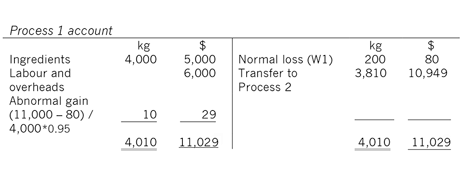Workings

Here we need to calculate the scrap value. The value of units transferred to Process 2 is a balancing figure.

1. Number of kg of normal loss scrap amount per kg = 200 x 0.4 = \$80 [Dr Scrap A / C \$80, Cr Process A / C \$80]

Be careful here! The scrap value also affects the abnormal gain or loss accounts. Since the staff didn’t eat the number of bars that they were entitled to, the scrap value (the 40c per bar) is lower than 200 40c. In fact, it is 10 x 40c = \$4 lower (the abnormal gain). This needs to be reflected in the scrap account and the abnormal gain account.Work in progress example
Assuming at the month end there are now part-completed bars (work- in-progress). Assuming also that he stopped charging staff for the bars that they had eaten. The data for Process 2 was as follows:For questions that include WIP, we need to calculate equivalent units. First, we need to choose the method of valuing WIP. In an exam, use the first in first out (FIFO) method if the percentage completion of each element of opening WIP is given. Use the weighted average (WA) method if the value of each element of opening WIP is given. [Note that the two methods give different valuations for the closing WIP.]

In the weighted average method, no distinction is made between units of opening inventory and new units introduced to the process during the accounting period.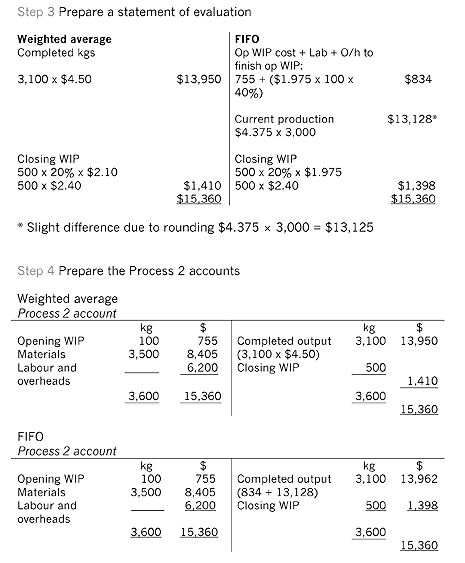Heather Freer is management accounting technical author at BPP Learning Media